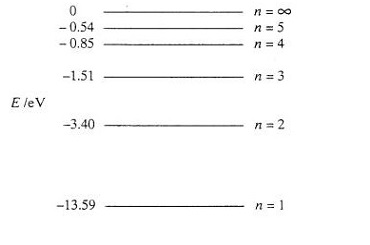Energy Level and Energy Band

ENERGY LEVELS

Near the nucleus, electrons are bound more strongly than those further away from the nucleus. The energy required to pull an electron out of its orbit is higher in the inner orbit than in the outer orbit. As a result, energy levels are used to refer to orbits. The number of electrons that can be received or sent away from an element's outermost orbit to another element in a reaction determines its valency. The valence electrons of elements with three or fewer valence electrons are given away, but elements with five or more valence electrons receive the balance electrons to make up the total of eight for stability. Valence electrons are electrons in the outermost orbit that are weakly connected to the nucleus. In the outermost orbit of a stable atom, there are 8 valence electrons, while in the outermost orbit of an unstable atom, there are less than 8 valence electrons.

The energy of Electron:

Consider the hydrogen atom, which has only one electron in its orbit. When the nucleus' electrostatic force equals the electron's centrifugal force, the system is stable. The energy level grows in inverse proportion to the square of the orbit number as the orbit number increases. The greater the forces that attach an electron to the nucleus get as it gets closer. The energy level indicates how much energy would be required to remove an electron from its orbit or shell.

The amount of energy required to carry one electron via a potential difference of one volt (1 eV = 1.60 x 10-19 J) is defined as an electron volt (eV). The energy levels that electrons may occupy appear as hands. Within any given substance, there are two separate bands in which electrons can exist. The valence band and the conduction hand are the two. The energy gap that separates these two bands is known as the forbidden gap, because no electrons may exist within it. The identical energy band diagram is shown in Figure.Energy Level of an electron in Hydrogen Atom

The forbidden gap is wide when the number of electrons in the outermost orbit exceeds four, and the material is categorized as an insulator. The amount of additional energy necessary for the electrons to escape the orbit in an insulator must be considered for them to go to the conduction band. When the number of electrons in the outermost orbit is less than four, detaching the electrons from the orbit with a modest amount of energy is easy, and the material is designated as a conductor. The number of electrons in the outermost orbit of a semi-conductor is equal to four, therefore it can behave either as a conductor or as an insulator. In general, the total energy required to remove an electron is proportional to the material's resistivity. The resistivity increases as the energy required to detach the outermost electron increases, and vice versa.